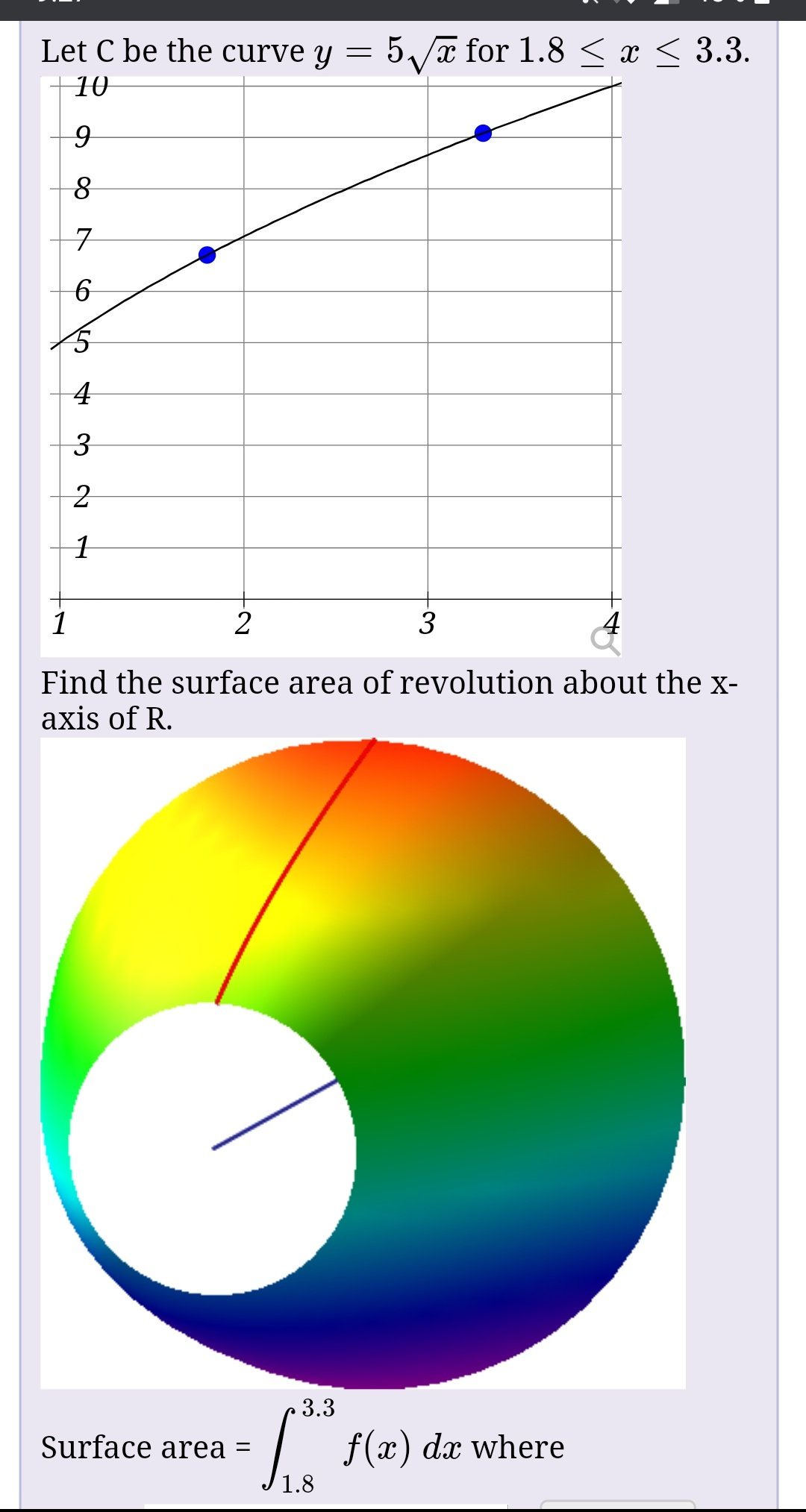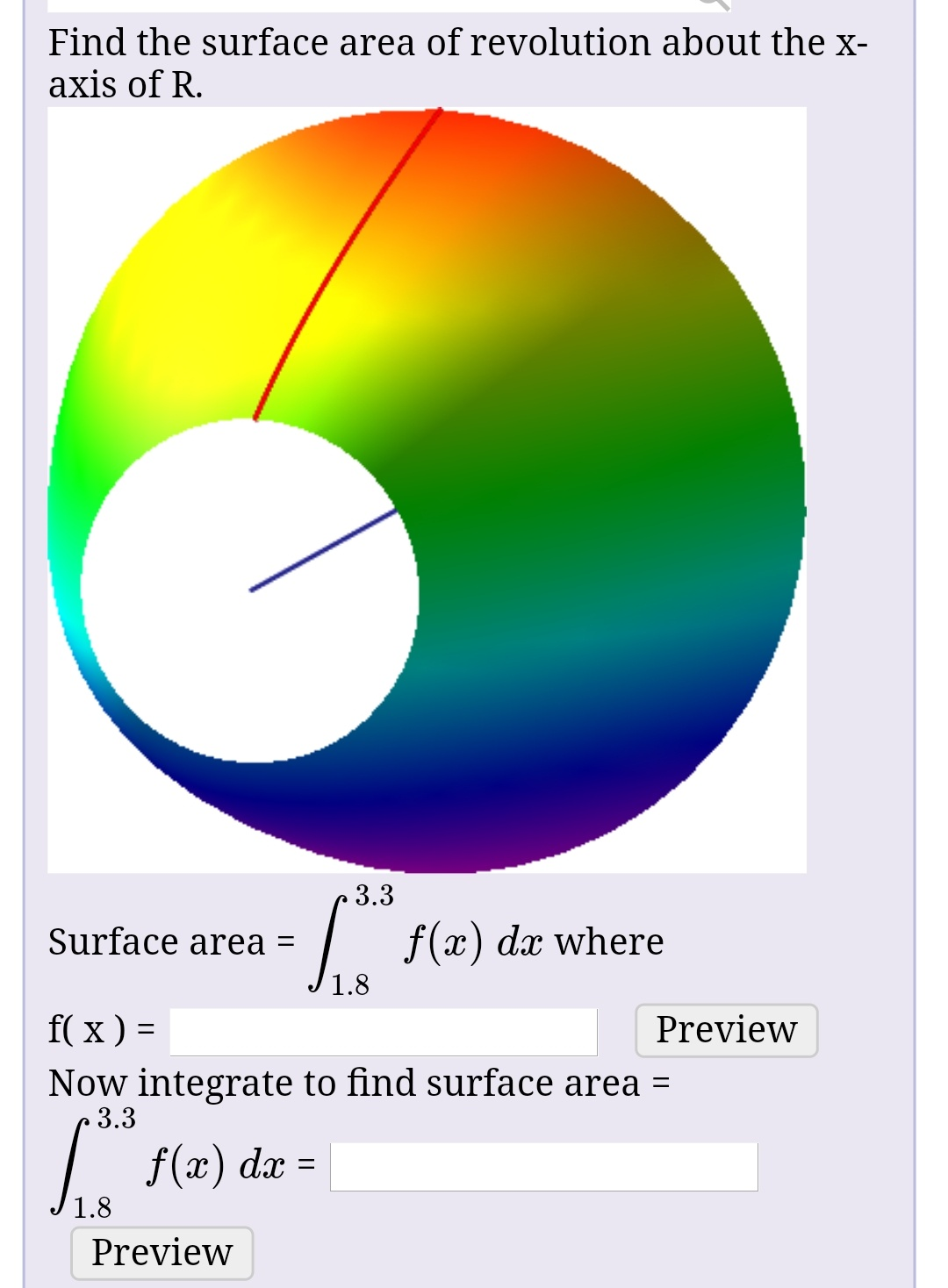# Let C be the curve y = 5/a for 1.8 < x < 3.3.103Find the surface area of revolution about the x-axis of R.3.3f(x)Surface area =dx where1.8 Find the surface area of revolution about the x-axis of R.3.3| f(x) dæ whereSurface area =1.8f( x ) =PreviewNow integrate to find surface area =3.3f(x) dx =1.8Preview

Question
8 viewshelp_outlineImage TranscriptioncloseLet C be the curve y = 5/a for 1.8 < x < 3.3. 10 3 Find the surface area of revolution about the x- axis of R. 3.3 f(x) Surface area = dx where 1.8 fullscreenhelp_outlineImage TranscriptioncloseFind the surface area of revolution about the x- axis of R. 3.3 | f(x) dæ where Surface area = 1.8 f( x ) = Preview Now integrate to find surface area = 3.3 f(x) dx = 1.8 Preview fullscreen
check_circle

star
star
star
star
star
1 Rating
Step 1

Given:

Step 2

Calculation:

Step 3

Step 2 as fo...

### Want to see the full answer?

See Solution

#### Want to see this answer and more?

Solutions are written by subject experts who are available 24/7. Questions are typically answered within 1 hour.*

See Solution
*Response times may vary by subject and question.
Tagged in

### Other